Next: Drift Equations Up: Plasma Fluid Theory Previous: Cold-Plasma Equations

# MHD Equations

The MHD equations take the form: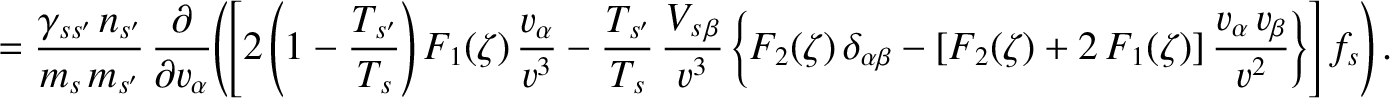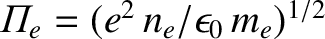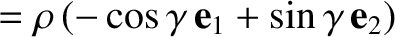(377)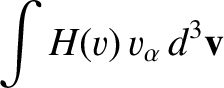(378)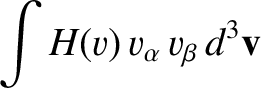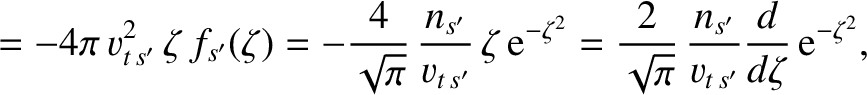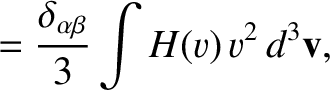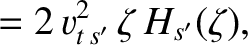(379)

and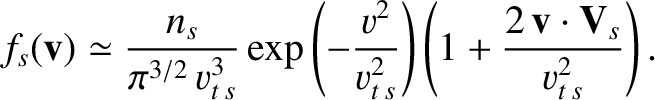(380)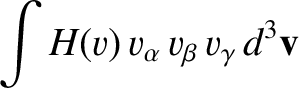(381)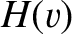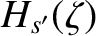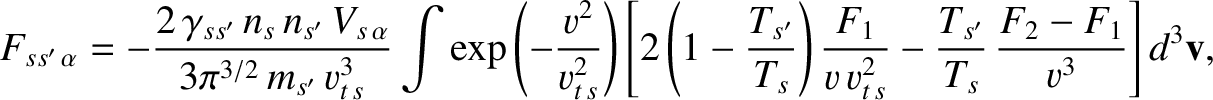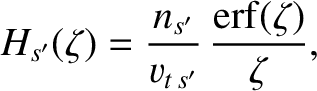(382)

These equations can also be simplified by making use of the smallness of the mass ratio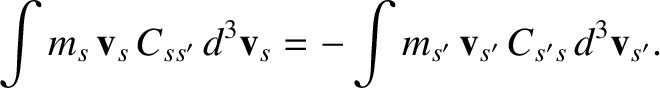. Now, according to the ordering adopted in Sect. 3.9,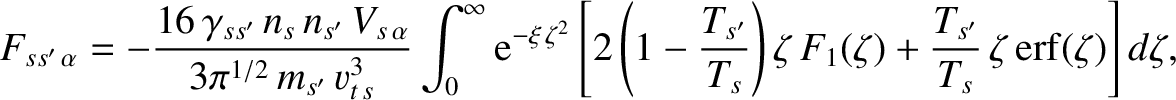in the MHD limit. It follows from Eqs. (372) and (373) that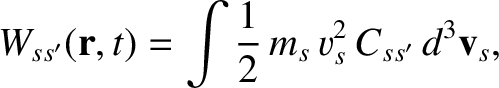(383)

and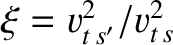(384)

The main point, here, is that in the MHD limit the velocity difference between the electron and ion fluids is relatively small.

Equations (377) and (380) yield the continuity equation: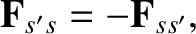(385)

where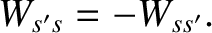.

Equations (378) and (381) can be summed to give the equation of motion: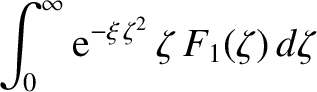(386)

Here,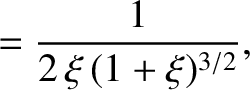is the total pressure. Note that all terms in the above equation are the same order in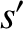.

The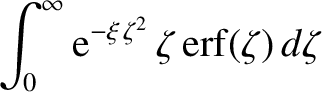components of Eqs. (378) and (381) yield the Ohm's law: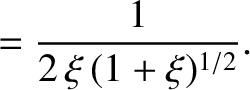(387)

This is sometimes called the perfect conductivity equation, since it is identical to the Ohm's law in a perfectly conducting liquid.

Equations (379) and (382) can be summed to give the energy evolution equation: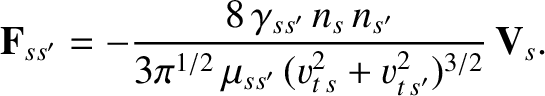(388)

Equations (385) and (388) can be combined to give the more familiar adiabatic equation of state: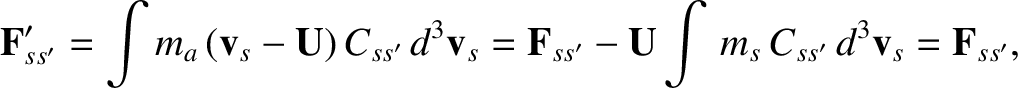(389)

Finally, thecomponents of Eqs. (379) and (382) yield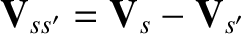(390)

or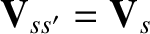[see Eq. (273)]. Thus, we expect equipartition of the thermal energy between electrons and ions in the MHD limit.Next: Drift Equations Up: Plasma Fluid Theory Previous: Cold-Plasma Equations
Richard Fitzpatrick 2011-03-31